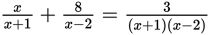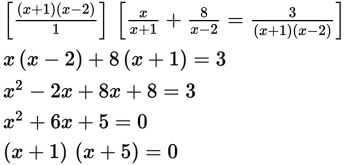# SAT Math Multiple Choice Question 900: Answer and Explanation

### Test Information

Question: 900

15. What is/are the solution(s) to the equation?

• A. -5 only
• B. -1 and -5
• C. 1 and 5
• D. No real solution

Explanation:

A

Difficulty: Medium

Category: Passport to Advanced Math / Exponents

Strategic Advice: When solving a rational equation that has more than two terms, a good strategy is to eliminate the fractions first by multiplying both sides of the equation by the least common denominator. Don't forget to check for extraneous solutions (solutions that result in a denominator equal to 0) before selecting your final answer.

Getting to the Answer: Multiply both sides of the equation by the common denominator, (x + 1)(x - 2), to eliminate the fractions. Once complete, solve for x by factoring:Setting each factor equal to 0 and solving gives x = -1 and x = -5. Now, take another look at the denominators in the original equation. The equation is undefined when x is -1 or 2, so one of the solutions you found (-1) is extraneous. Therefore, -5 is the only valid solution, making (A) the correct answer.